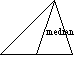index: click on a letter A B C D E F G H I J K L M N O P Q R S T U V W X Y Z A to Z index index: subject areas numbers & symbols sets, logic, proofs geometry algebra trigonometry advanced algebra & pre-calculus calculus advanced topics probability & statistics real world applications multimedia entrieswww.mathwords.com about mathwords website feedback

 Median of a Triangle A line segment drawn from one vertex to the midpoint of the opposite side. Note: The three medians of a triangle are concurrent, intersecting at the centroid.See also